# 9 Inductance 1 Mutual inductance 1 2 I

• Slides: 8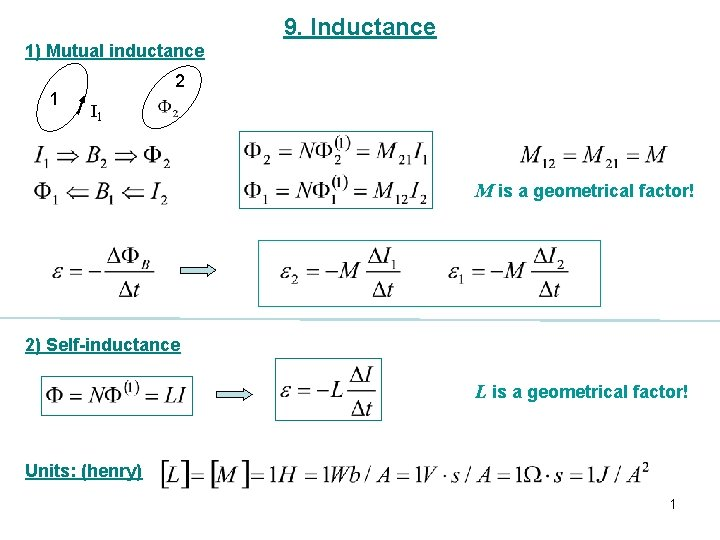9. Inductance 1) Mutual inductance 1 2 I 1 M is a geometrical factor! 2) Self-inductance L is a geometrical factor! Units: (henry) 1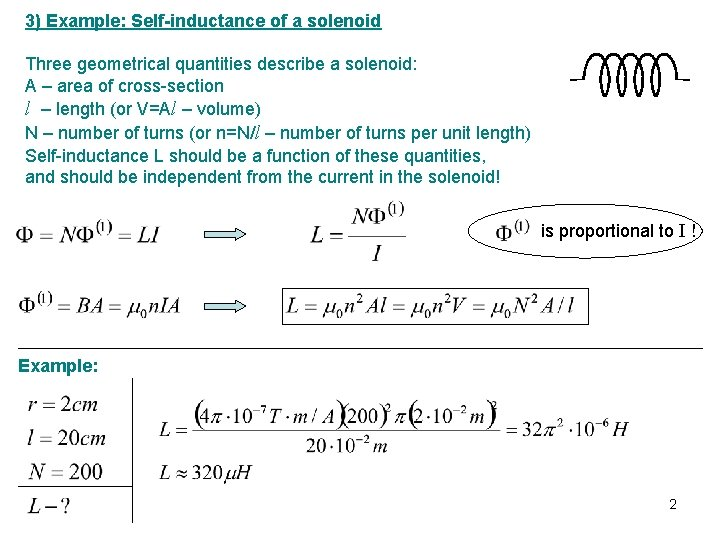3) Example: Self-inductance of a solenoid Three geometrical quantities describe a solenoid: A – area of cross-section l – length (or V=Al – volume) N – number of turns (or n=N/l – number of turns per unit length) Self-inductance L should be a function of these quantities, and should be independent from the current in the solenoid! is proportional to I ! Example: 210. RL circuits 1) Circuit with emf I R L Relaxation time 2) Circuit without emf 3Example: Two tightly wound solenoid have the same length and circular crosssectional area. They use wires made from the same material, but solenoid 1 uses wire that is half as thick as solenoid 2. What is the ratio of their inductances? What is the ratio of their time constants (assuming no other resistance in the circuits)? a) b) 4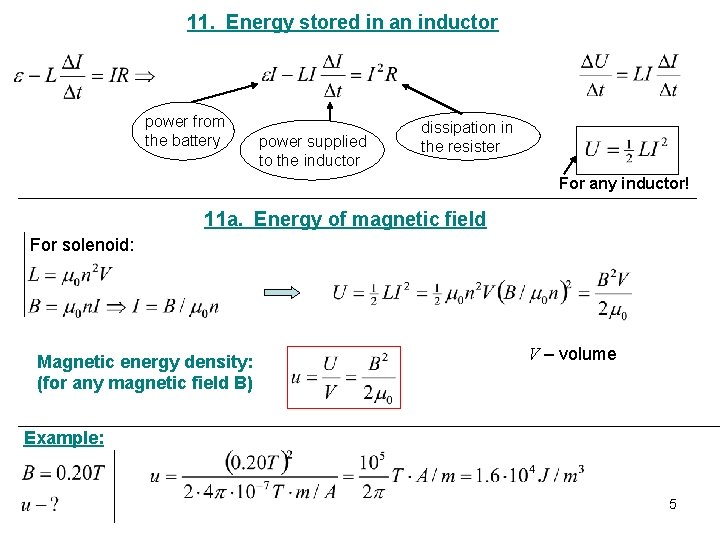11. Energy stored in an inductor power from the battery power supplied to the inductor dissipation in the resister For any inductor! 11 a. Energy of magnetic field For solenoid: Magnetic energy density: (for any magnetic field B) V – volume Example: 5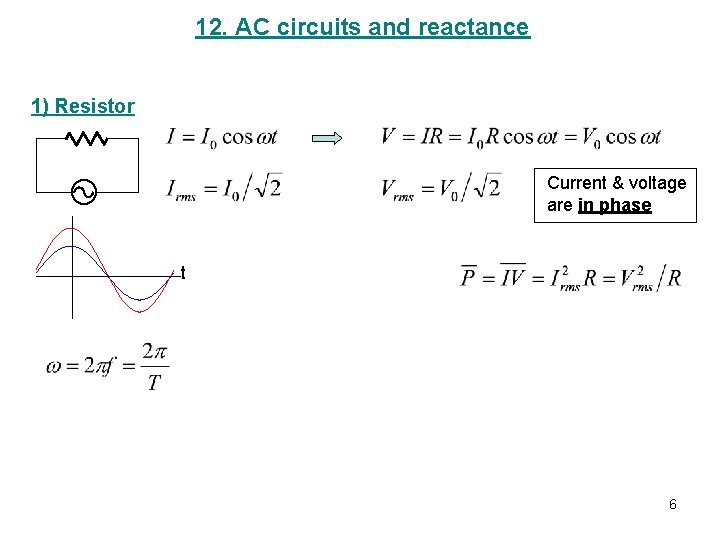12. AC circuits and reactance 1) Resistor Current & voltage are in phase t 6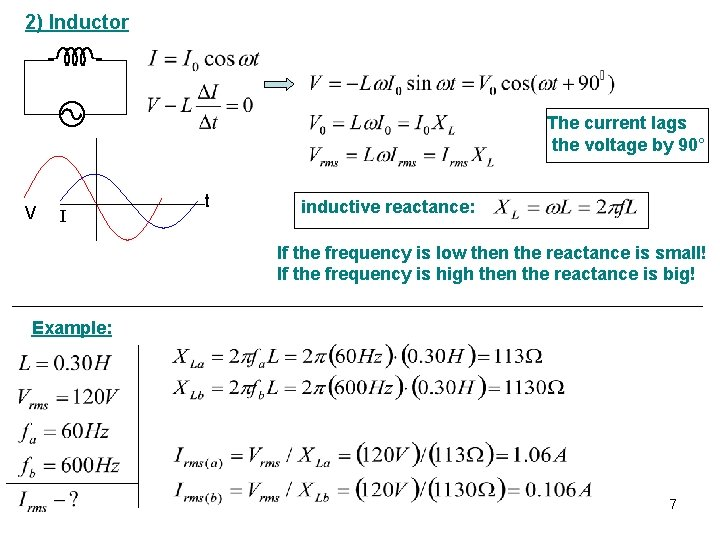2) Inductor The current lags the voltage by 90° V I t inductive reactance: If the frequency is low then the reactance is small! If the frequency is high then the reactance is big! Example: 7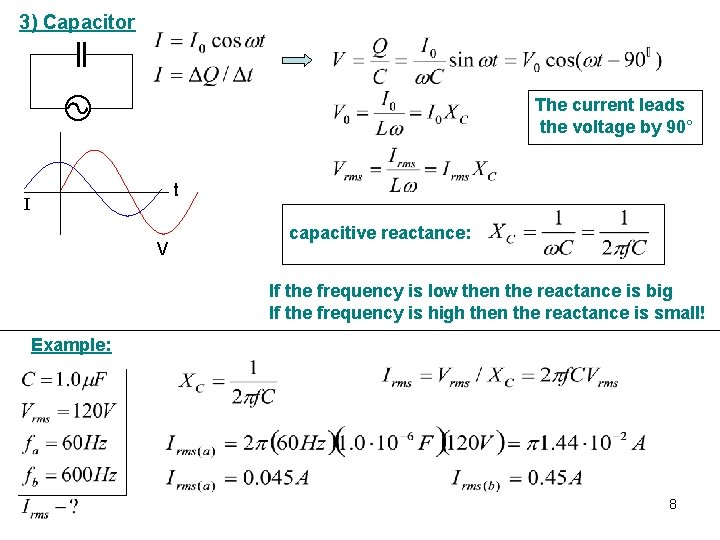3) Capacitor The current leads the voltage by 90° t I V capacitive reactance: If the frequency is low then the reactance is big If the frequency is high then the reactance is small! Example: 8